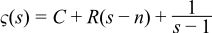#Boost C++ Libraries

...one of the most highly regarded and expertly designed C++ library projects in the world.

This is the documentation for an old version of boost. Click here for the latest Boost documentation.

#### Riemann Zeta Function

##### Synopsis

```#include <boost/math/special_functions/zeta.hpp>
```

```namespace boost{ namespace math{

template <class T>
calculated-result-type zeta(T z);

template <class T, class Policy>
calculated-result-type zeta(T z, const Policy&);

}} // namespaces
```

The return type of these functions is computed using the result type calculation rules: the return type is `double` if T is an integer type, and T otherwise.

The final Policy argument is optional and can be used to control the behaviour of the function: how it handles errors, what level of precision to use etc. Refer to the policy documentation for more details.

##### Description
```template <class T>
calculated-result-type zeta(T z);

template <class T, class Policy>
calculated-result-type zeta(T z, const Policy&);
```

Returns the zeta function of z:##### Accuracy

The following table shows the peak errors (in units of epsilon) found on various platforms with various floating point types, along with comparisons to the GSL-1.9 and Cephes libraries. Unless otherwise specified any floating point type that is narrower than the one shown will have effectively zero error.

Table 44. Errors In the Function zeta(z)

Significand Size

Platform and Compiler

z > 0

z < 0

53

Win32, Visual C++ 8

Peak=0.99 Mean=0.1

GSL Peak=8.7 Mean=1.0

Cephes Peak=2.1 Mean=1.1

Peak=7.1 Mean=3.0

GSL Peak=137 Mean=14

Cephes Peak=5084 Mean=470

64

RedHat Linux IA_EM64, gcc-4.1

Peak=0.99 Mean=0.5

Peak=570 Mean=60

64

Redhat Linux IA64, gcc-4.1

Peak=0.99 Mean=0.5

Peak=559 Mean=56

113

HPUX IA64, aCC A.06.06

Peak=1.0 Mean=0.4

Peak=1018 Mean=79

##### Testing

The tests for these functions come in two parts: basic sanity checks use spot values calculated using Mathworld's online evaluator, while accuracy checks use high-precision test values calculated at 1000-bit precision with NTL::RR and this implementation. Note that the generic and type-specific versions of these functions use differing implementations internally, so this gives us reasonably independent test data. Using our test data to test other "known good" implementations also provides an additional sanity check.

##### Implementation

All versions of these functions first use the usual reflection formulas to make their arguments positive:The generic versions of these functions are implemented using the series:for large z, and using the globally convergent series:In all other cases. The crossover point for these is chosen so that the first series is used only if it will converge reasonably quickly, the problem with this series is that convergence become slower the more terms you take, so we really do have to be certain of convergence before using this series, even though the alternative is often quite slow.

When the significand (mantissa) size is recognised (currently for 53, 64 and 113-bit reals, plus single-precision 24-bit handled via promotion to double) then a series of rational approximations devised by JM are used.

For 0 < z < 1 the approximating form is:For a rational approximation R(1-z) and a constant C.

For 1 < z < 4 the approximating form is:For a rational approximation R(n-z) and a constant C and integer n.

For z > 4 the approximating form is:

ζ(z) = 1 + eR(z - n)

For a rational approximation R(z-n) and integer n, note that the accuracy required for R(z-n) is not full machine precision, but an absolute error of: ε/R(0). This saves us quite a few digits when dealing with large z, especially when ε is small.